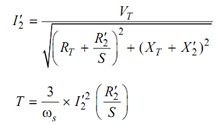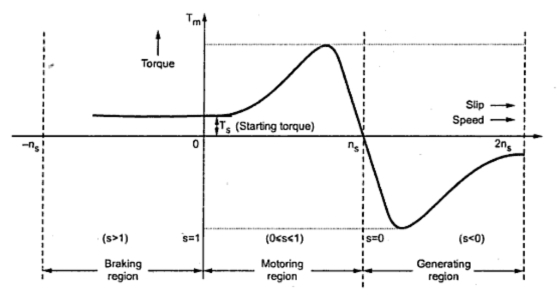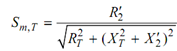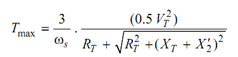## Torque-Slip Characteristics Assignment Help

Assignment Help: >> Induction Motors - Torque-Slip Characteristics

Torque-Slip (T-S) Characteristics:

For the better expression of the torque make thevenin's equivalent circuit for the left side of the terminal 1-2.

The thevenin impedance.

Z¯T  = (R1 + j X1 ) | | j X m  = RT  + j XT

Thevenin voltage

T  = V1 ( j X m / ( R1 + j X1 + j X m ))Substitute the value of I2′ in Eq.

T =  3/ ωs  .  (V T) 2 (R2′ /S) /(RT +( R2′/S)2 +(XT + X2 ′)2

Eq. (57) expresses the torque as a function of voltage and slip. This is seen that for a fixed value of slip, torque is equal to proportional to the square of voltage. Figure illustrates the torque slip characteristics of an induction motor for the rated voltage.Figure: Torque-Slip Characteristics

The torque is zero While slip is zero; (Nr = Ns). We have already discussed that induction motor torque is zero at synchronous speed. At slip Sm, T; the motor gives the maximum torque that is also called as break down torque. While the rotor is stand still (S = 1), the torque corresponds to beginning torque Ts. In normally designed motor Ts is much less than Tmax (Break down torque). The torque slip characteristics from no-load to rather beyond full-load are almost linear.

The condition for maximum torquewhere SM, T is the value of slip at which maximum torque occurs, and the maximum torque is thenFrom the Eq. (59), we came to the conclusion that the maximum value of the torque does not based upon rotor resistance ( R2′ ) .

The slip at which maximum torque occurs indirectly proportional to the rotor resistance ( R2′ ) . At S = 1, we have the beginning torque which increases by adding resistance in the rotor circuit.#### Assured A++ Grade

Get guaranteed satisfaction & time on delivery in every assignment order you paid with us! We ensure premium quality solution document along with free turntin report!

All rights reserved! Copyrights ©2019-2020 ExpertsMind IT Educational Pvt Ltd